# Movement

From the crossing of two perpendicular roads started two cyclists (each at the different road). One runs at average speed 28 km/h, the second at average speed 24 km/h. Determine the distance between them after 45 minutes cycling.

Correct result:

x =  27.6586 km

#### Solution: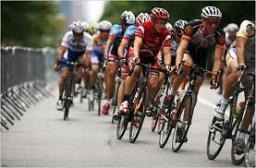We would be pleased if you find an error in the word problem, spelling mistakes, or inaccuracies and send it to us. Thank you!Tips to related online calculators
Do you want to convert length units?
Do you want to convert velocity (speed) units?
Do you want to convert time units like minutes to seconds?
Pythagorean theorem is the base for the right triangle calculator.

#### You need to know the following knowledge to solve this word math problem:

We encourage you to watch this tutorial video on this math problem:

## Next similar math problems:

• Two cyclistsTwo cyclists started from crossing in the same time. One goes to the north speed 20 km/h, the second eastward at speed 26 km/h. What will be the direct distance cycling 30 minutes from the start?From the junction of two streets that are perpendicular to each other, two cyclists (each on another street) walked out. One ran 18 km/h and the second 24 km/h. How are they away from a) 6 minutes, b) 15 minutes?
• Two aircraftTwo planes fly to the airport. At some point, the first airplane is away from the airport 98 km and the second 138 km. The first aircraft flies at an average speed of 420 km/h, the second average speed is 360 km/h, while the tracks of both planes are perp
• Motion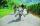Cyclist started at 9:00 from point S to point T. After 10 minutes, followed him at the same speed the second cyclist. Walker, which went from T to S, started at 9:35. After 71 minutes he met the first cyclist and after next 8 minutes the second cyclist. D
• Two cars 2Two cars started from two positions 87 km distant at the same time in opposite directions at speeds 81 km/h and 75 km/h. What was the distance between them after 2 hours 50 minutes of driving.Passenger car and an ambulance come to the rectangular crossroad, the ambulance left. Passenger car at speed 39 km/h and ambulance at 41 km/h. Calculate such a relative speed of the ambulance move to the car.
• Cars speedsDistance from city A to city B is 108 km. Two cars were simultaneously started from both places. The speed of a car coming from city A was 2 km/h higher than the second car. What was the speed of each car if they met in 54 minutes?
• Two trains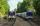Two trains go at each other. First at speed 40 km/h and the second at speed 60 km/h. At what distance will be 30 minutes before they will meet?
• Peleton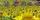Cycling race was run at an average speed of 45 km/h. One cyclist lost with defect 8 minutes. How long and how far he must go at speed 51 km/h to catch peleton again?
• Two citiesThe distance between cities A and B is 132 km. At 9.00 am, the cyclist started the bike at an average speed of 24 km/h, and at 10.00 h started from the B cyclist at an average speed of 30 km/h. How long and far from A will they both meet?
• Two aircraftFrom the airport will start simultaneously two planes, which fly tracks are perpendicular to each other. The first flying speed of 680 km/h and the second 840 km/h. Calculate how far the aircraft will fly for half an hour.
• Two parallel chordsIn a circle 70 cm in diameter, two parallel chords are drawn so that the center of the circle lies between the chords. Calculate the distance of these chords if one of them is 42 cm long and the second 56 cm.
• Pedestrian and the cyclist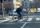Pedestrian and cyclist went against each from the two cities that are distant 40 km. Pedestrian started 30 minutes before the cyclist and walk an average speed of 5 km/h. What speed went cyclist when met after 120 minutes from the start of the pedestrian?
• RectangleThe rectangle is 21 cm long and 38 cm wide. Determine the radius of the circle circumscribing rectangle.
• MinuteTwo boys started from one place. First went north at velocity 3 m/s and the second to the east with velocity 4 m/s. How far apart they are after minute?
• Two peopleTwo straight lines cross at right angles. Two people start simultaneously at the point of intersection. John walking at the rate of 4 kph in one road, Jenelyn walking at the rate of 8 kph on the other road. How long will it take for them to be 20√5 km apa
• Tourists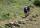From the cottage started first group of tourists at 10:00 AM at speed 4 km/h. The second started after them 47 minutes later at speed 6 km/h. How long and how many kilometers from the cottage will catch the first group?